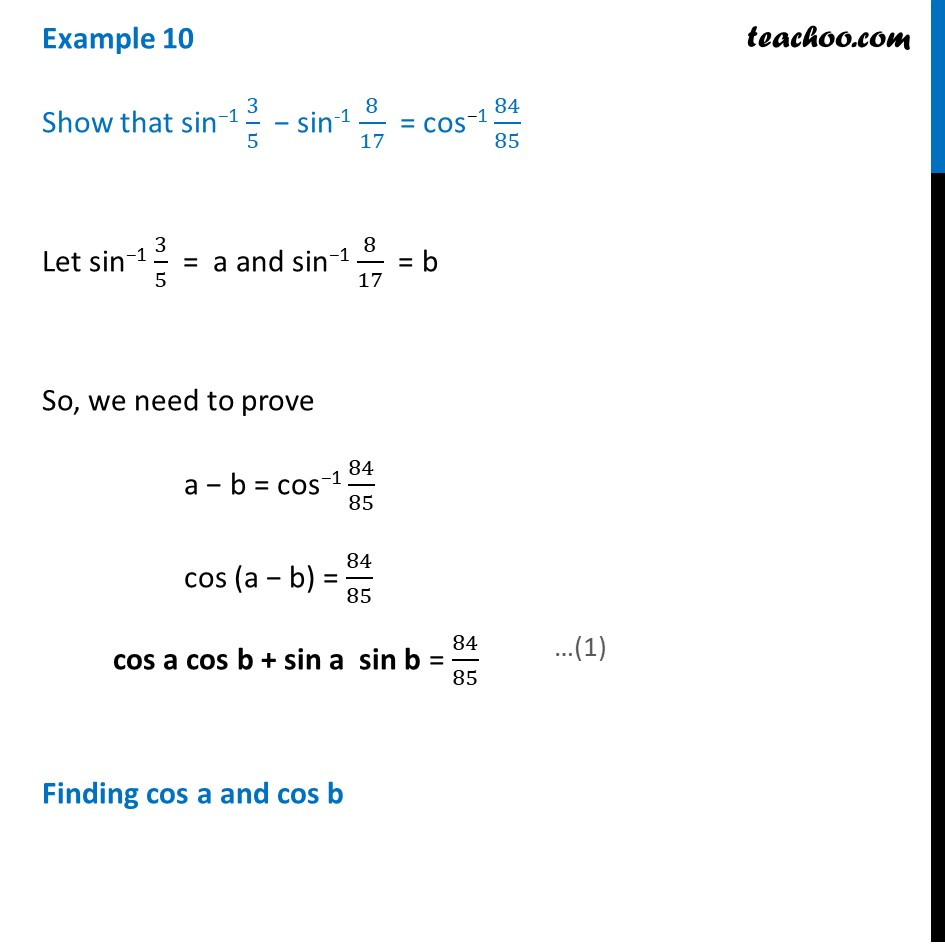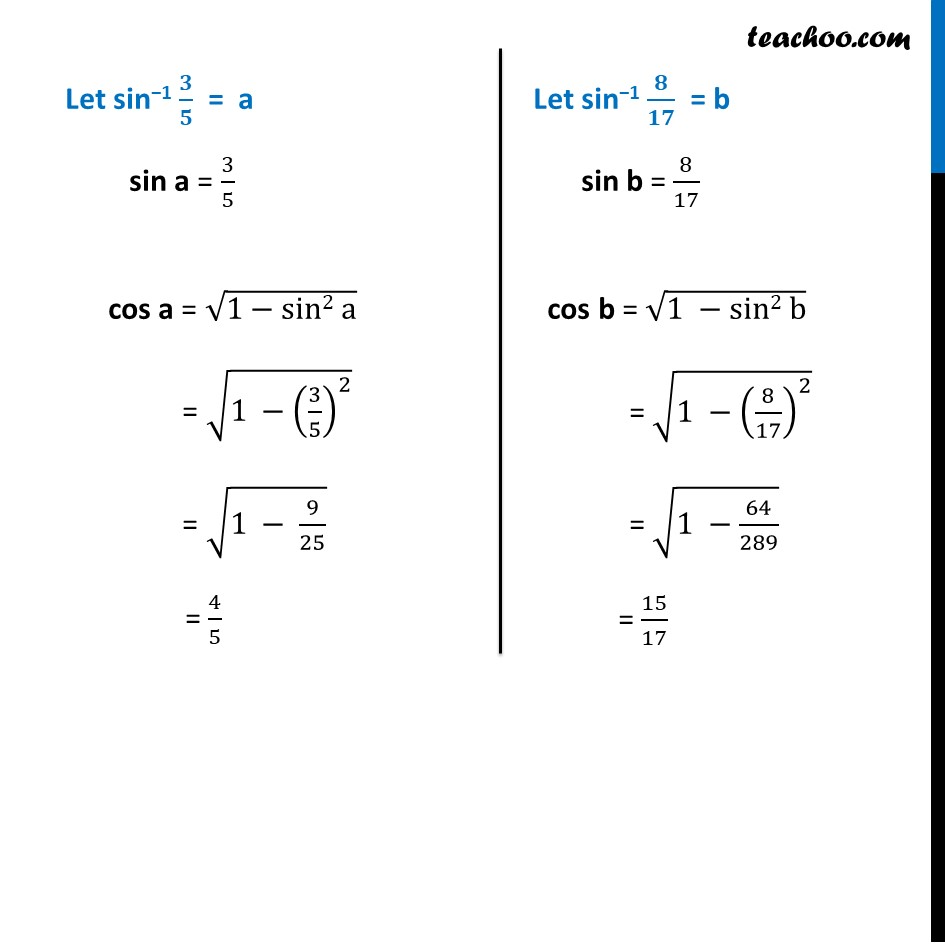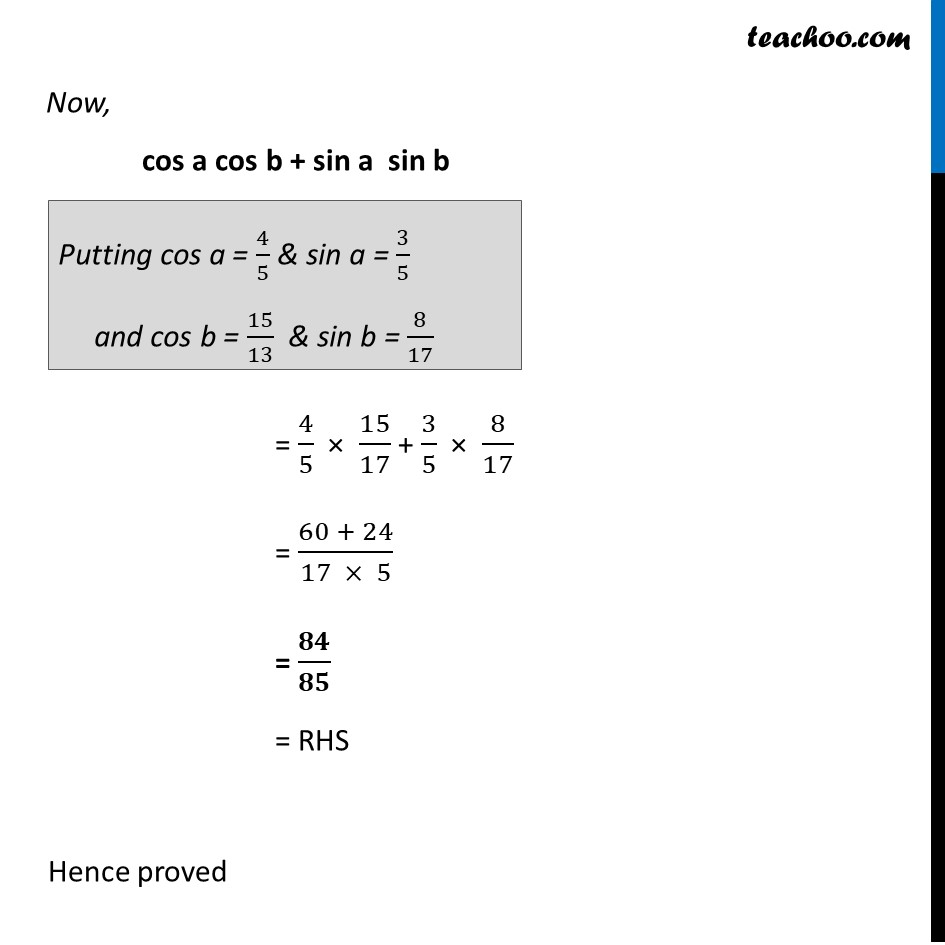Examples

Chapter 2 Class 12 Inverse Trigonometric Functions
Serial order wiseLearn in your speed, with individual attention - Teachoo Maths 1-on-1 Class

### Transcript

Question 4 Show that sin−1 3/5 − sin-1 8/17 = cos−1 84/85 Let sin−1 3/5 = a and sin−1 8/17 = b So, we need to prove a − b = cos−1 84/85 cos (a − b) = 84/85 cos a cos b + sin a sin b = 84/85 Finding cos a and cos b Let sin−1 𝟑/𝟓 = a sin a = 3/5 cos a = √(1−sin2 a) = √(1 −(3/5)^2 ) = √(1 − 9/25) = 4/5 Let sin−1 𝟖/𝟏𝟕 = b sin b = 8/17 cos b = √(1 −sin2 b) = √(1 −(8/17)^2 ) = √(1 −64/289) = 15/17 Let sin−1 𝟖/𝟏𝟕 = b sin b = 8/17 cos b = √(1 −sin2 b) = √(1 −(8/17)^2 ) = √(1 −64/289) = 15/17 Now, cos a cos b + sin a sin b = 4/5 × 15/17 + 3/5 × 8/17 = (60 + 24)/(17 × 5) = 𝟖𝟒/𝟖𝟓 = RHS Hence proved Putting cos a = 4/5 & sin a = 3/5 and cos b = 15/13 & sin b = 8/17